Next: Covariance of the Continuity Up: Proof of Covariance Previous: Lorentz Boosts

## Spatial Inversion

We now consider the improper Lorentz transformation of reflection in space or the parity transformation: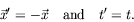(5.149)

We need to solve (5.122) for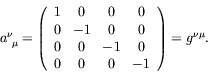(5.150)

We denote the Lorentz operator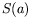by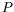for parity. Consider the following ansatz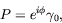(5.151)

where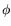is an arbitrary phase. Using equation 5.122, we have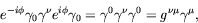(5.152)

as required.

In analogy to the proper Lorentz transformation for which a rotation of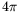reproduces the original spinors, we postulate that four space-inversions will reproduce the original spinors.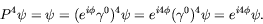(5.153)

Therefore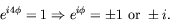(5.154)

We see that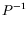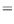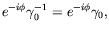(5.155)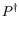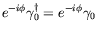(5.156)

and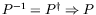is unitary.

The wave function thus transforms as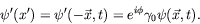(5.157)

In the nonrelativisitc limit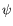approaches an eigenstate of. The positive- and negative-energy states at rest have opposite eigenvalues, or intrinsic parities. The intrinsic parity of a Dirac particle and antiparticle are opposite. This is to be contrasted to the Klein-Gordon case wherein one finds identical parities for the particle and antiparticle solutions.Next: Covariance of the Continuity Up: Proof of Covariance Previous: Lorentz Boosts
Douglas M. Gingrich (gingrich@ ualberta.ca)
2004-03-18